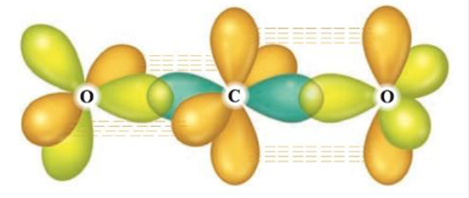Chapter 20, Problem 35E

Chapter
Section
Textbook Problem

# The following illustration shows the orbitals used to form the bonds in carbon dioxide.Each color represents a different orbital. Label each orbital, draw the Lewis structure for carbon dioxide, and explain how the localized electron model describes the bonding in CO2.

Interpretation Introduction

Interpretation: The orbitals that are used to form carbon dioxide (CO2) are shown. Each orbital is to be labeled. The Lewis structure of CO2 is to be drawn. The explanation of bonding in CO2 is to be stated on the basis of localized electron model.

Concept introduction: The bonding of any molecule is explained by localized electron model. The following steps are involved in this model,

• Lewis structure of the molecule is drawn.
• The arrangement of electron pairs is determined by using VSPER model.
• The hybrid orbital of each atom is specified.

To determine: The labeled diagram of orbitals to show the bonding in CO2 ; the Lewis structure of CO2 ; the explanation of bonding in CO2 on the basis of localized electron model.

Explanation

Explanation

In the orbital representation of CO2 , dotted line shows the orbital. The pie bond is formed due to lateral overlapping of orbitals whereas sigma bond is formed due to axial overlapping of orbitals. The labeled diagram of orbitals is,

Figure 1

The Lewis structure of CO2 is as follows.

The Lewis structure represents the arrangement of valence electrons.

The first step in determining the Lewis structure is to determine the number of valence electrons. The atomic number of central atom carbon (C) is 6 and its electronic configuration is,

1s22s22p2

The valence electron of carbon is 4 .

The atomic number of oxygen (O) is 8 and its electronic configuration is,

1s22s22p4

The valence electron of oxygen is 6

### Still sussing out bartleby?

Check out a sample textbook solution.

See a sample solution

#### The Solution to Your Study Problems

Bartleby provides explanations to thousands of textbook problems written by our experts, many with advanced degrees!

Get Started